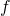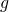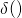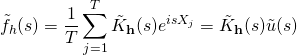# Fast Kernel Density Estimation using the Fast Fourier Transform

## 1. Setup

This Post is about how to speed up the computation kernel density estimators using the FFT (Fast Fourier Transform). Let bebe a random sample drawn from an unknown distribution with density. Remember, the kernel density estimator with bandwidthis then given by

(1)### 1.1 Convolution Theorem

In the following we will use the notation from a previous article where the convolution theorem for the Fourier Transform was introduced. Accordingly the discrete version of the convolution theorem is given by: Letandbe two functions defined at evenly spaced points, their convolution is given by:

(2)To speed up the kernel computation we will use a particular feature given by:

(3)### 1.2 Using DFFT Convolution to estimateSince the discrete convolution theorem requires functions observed at evenly spaced points we create a fine gridof lengthwhere we want to evaluate the density.

(4)whereis the dirac delta function. To derive the estimate for all pointsthe computer has to handleoperations.

Following , let the discrete Fourier transform ofbe denoted by. The Fourier transform of (4) foris then using convolution and translation given by

(5)whereis the Fourier transform of the data. The result corresponding to (4) can then be obtained by applying the inverse DFFT. All these steps then involve onlyoperations.is derived using a histogram on the observed data with binning boundaries defined by, and then applying the Fast Fourier transform.

### 1.3 Implementation

To implement the method the fft function that comes with the numpy package was chosen. The numpy fft() function will return the approximation of the DFT from 0 to. Therefore fftshift() is needed to swap the output vector of the fft() right down the middle. So the output of fftshift(fft()) is then fromto. The computation using the fft was around 5 times faster.

import numpy as np
import matplotlib.pyplot as plt
import timeit

# Simulation
N=500
X=np.random.normal(-0,1.5,N)

plt.scatter(np.linspace(0,N,N), X, c="blue")
plt.xlabel('sample')
plt.ylabel('X')
plt.show()

# Presetting
Nout=2**7

def epan_kernel(u,b):
u= u/b
return max(0, 1./b*3./4*(1-u**2))

# Usual estimation
def dens(s, X, h=0.5):
return [epan_kernel(s-x,1) for x in X]

start = timeit.default_timer()

grid =  np.linspace(-5, 5, num=Nout)
density=[(1./X.size)*sum( dens(y, X) ) for y in grid]
plt.plot(grid, density )

stop = timeit.default_timer()
print('Time: ', stop - start)

# Estimation using FFT
start = timeit.default_timer()

to = np.linspace(-5, 5, Nout+1)
t = grid
kernel=[epan_kernel(x,1) for x in t]
kernel_ft=np.fft.fft(kernel)
hist,bins=np.histogram(X, bins=to)
density_tmp=kernel_ft.flatten()*np.fft.fft(  hist  )
denisty_fft=(1./X.size)*np.fft.fftshift( np.fft.ifft(density_tmp).real)
plt.plot(t, denisty_fft)

stop = timeit.default_timer()
print('Time: ', stop - start)
print(np.sqrt(sum((density-denisty_fft)**2)))


### References

 B. W. Silverman, “Algorithm as 176: kernel density estimation using the fast fourier transform,” Journal of the royal statistical society. series c (applied statistics), vol. 31, iss. 1, p. 93–99, 1982.
[Bibtex]
@article{Silverman1982,
ISSN = {00359254, 14679876},
URL = {http://www.jstor.org/stable/2347084},
author = {B. W. Silverman},
journal = {Journal of the Royal Statistical Society. Series C (Applied Statistics)},
number = {1},
pages = {93--99},
publisher = {[Wiley, Royal Statistical Society]},
title = {Algorithm AS 176: Kernel Density Estimation Using the Fast Fourier Transform},
volume = {31},
year = {1982}
}

### 1 thought on “Fast Kernel Density Estimation using the Fast Fourier Transform”

This site uses Akismet to reduce spam. Learn how your comment data is processed.